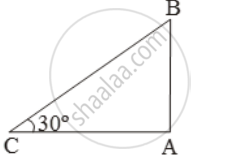# When the sun is 30º above the horizontal, Find the length of shadow cast by a building 50m high - Mathematics

Sum

When the sun is 30º above the horizontal, Find the length of shadow cast by a building 50m high

#### Solution

Let AB be the building and AC be its shadow.

Then, AB = 50 m and θ = 30º.\therefore \frac{AC}{AB}=\text{cot 3}0^\text{o}=\sqrt{3}

\Rightarrow \frac{AC}{50}=\sqrt{3}

⇒ AC = 50√3 cm.

Concept: Heights and Distances
Is there an error in this question or solution?